# Short Notes: Electrical Conduction# Electric Current

The electric current is produced by the flow of electric charges. The electric current is defined as the rate of flow of charge. If a charge q flows in time t, then electric current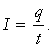If small charge dq flows in time dt, then instantaneous current,The S.I. unit of current is coulomb/second called ampere denoted by (A).

Conventionally the direction of current is taken along the direction of flow of positive charge and opposite to the direction of flow of negative charge.

Though electric current has direction but it is a scalar quantity because it does not obey the laws of vector addition.

# Drift Velocity and Mobility

The charge carriers in a metallic conductor are free electrons. When no potential difference is applied, the motion of free electrons is random, so there is no net current in any direction.

When a potential difference is applied across a conductor, the free electrons collide with positive ions/atoms of crystal lattice but between two collisions they gain a small average velocity in the direction of accelerating potential. This small average velocity is called the drift velocity.

The direction of current is opposite to that of drift velocity.

Ifis the electric field due to applied potential difference, then acceleration of electronwhere e is charge and m is mass of electron.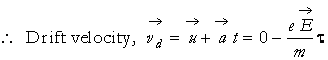Where τ is time between two successive collisions.

Since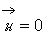due to random motion of electron.where λ = mean free path

v = root mean square velocity of electrons.

Mobility : The mobility of a charged particle is defined as the drift velocity per unit electron field, i.e.,# Relation between Drift velocity and Electric Current

The electric current in a metallic conductor is produced due to drift of electrons opposite to the direction of electric field. The relation between current (i) and drift velocity  (vd) is i = neAvd

where n = number of free electrons per cubic metre.

A = cross-sectional area of conductor.

# Ohm`s Law

When a potential difference is applied across a conductor, the current begins to flow through it. According to Ohm?s law, the electric current produced in a conductor is directly proportional to the potential difference applied across it provided the physical conditions like pressure, temperature, etc. remain uncharged.

i.e.,   I  ∝ V

or   V ∝ I

⇒ V = IR

where R is a constant called electrical resistance or simply the resistance of the conductor.

The unit of electrical resistance is volt/ampere or ohm (denoted by Ω)

# Electrical Resistance

The hindrance offered by a conductor to the flow of current is called the electrical resistance. The resistance of a conductor depends on its length, cross-sectional area A and nature of material at a given temperature and is given by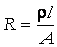where ρ is the resistivity of the material.

# V-I Characteristics

Linear and Non-Linear Ohmic and Non-ohmic Conductors : The conductors or circuit elements for which V-I graph is linear are called ohmic conductors. The examples are metallic conductors.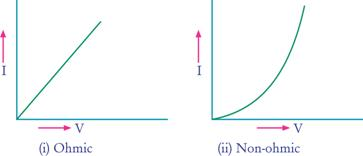On the other hand the circuit elements for which V-I graph is non-linear are called non-ohmic conductors. The examples are junction diodes and transistors.

# Electrical Energy

The electrical energy consumed (or heat produced) in a current carrying conductor of resistance R in time t is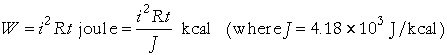This is called Joule’s law of heating.

If V is the potential difference across wire, then electrical energy consumed# Power

Rate of energy dissipation in a resistor is called the power, i.e.,# Resistivity (or Specific Resistance)

From relation,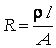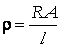If l = 1 m, A = 1 m, ρ = R.

That is, resistivity of a material is numerically equal to the resistance offered by the wire of that material of length 1 metre and cross-sectional area 1 square-metre.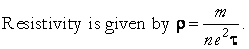The resistivity (ρ) depends only on material and is independent of dimensions at a given temperature. The S.I. unit of resistivity is ohm _ metre abbreviated as (Ωm).

# Conductance and Conductivity

The reciprocal of resistance is called the conductance (G)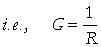Its S.I. unit is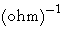or mho or seimen (S).

The reciprocal of resistivity is called the conductivity σ.# Colour Code for Carbon Resistances

Very high resistances are made of carbon. The value of high resistance is specified by four bands of different colours. The first three bands represent value of resistance while the last band represents tolerance. The first band represents first digit, second band represents second digit and third band represents multiplier in powers of 10. The colour of fourth band tells the tolerance. Absence of fourth band means a tolerance of 20%. The following table gives the colour code for carbon resistances.

 First letter of colour Colour Figure Multiplier % Tolerence B Black 0 100 B Brown 1 101 R Red 2 102 O Orange 3 103 Y Yellow 4 104 G Green 5 105 B Blue 6 106 V Violet 7 107 G Grey 8 108 W White 9 109 Gold ? 10-1 5 Silver ? 10-2 10 No colour ? ? 20

To memorise these colour codes, the following sentence is of great help.

B.B. ROY (of) Great Britain (has) Very Good Wife.

# Resistances in Series and Parallel

(i) When resistances are connected in series, the net resistance (Rs) is given by

R = R1 + R2 + R+……+ Rn

In Series I1= I=I(same)

voltage ,  Vs = V1 + V2 + V+……+ Vn

(ii) When resistances are connected in parallel, the net resistance (RP) is given by# Temperature Dependence of Resistance

The resistance of a metallic conductor increases with increase of temperature while resistance of a semiconductor decreases with increase of temperature and is given by

Rt = R0(  1 + αt)

where R0 is resistance at 0?C and Rt is resistance at t°C and α is temperature coefficient of resistance. In general if variation of temperature is not too large, then## Super Conductors

Some substance lose their resistance when cooled below a certain temperature. These substances are called superconductors and the temperature below which they lose resistance is called transition temperature. The transition temperature of Hg is 4.2 K.